Courses

# Quadrilaterals - General Geometry Mathematics Notes | EduRev

## Mathematics : Quadrilaterals - General Geometry Mathematics Notes | EduRev

The document Quadrilaterals - General Geometry Mathematics Notes | EduRev is a part of the Mathematics Course Additional Topics for IIT JAM Mathematics.
All you need of Mathematics at this link: Mathematics

Angles
It is common knowledge that the sum of the angles in a triangle is 180° but how about in polygons with a greater numbers of angles? If we are given a convex polygon with n sides and S is the sum of the measures of the interior angles then S = 180(n - 2).
Example
Find the sum of the measures of the interior angles in an octagon.
The octagon has 8 sides and we plug this value into our formula:
S = 180(8 - 2) = 1080°
Hence the sum of the measures of the interior angles in an octagon is 1080°.
Another thing with convex polygons is that the sum of the measures of the exterior angles is always 360°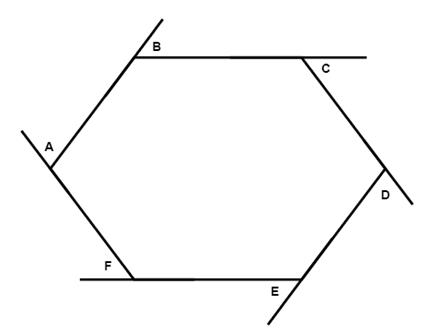m∠A + m∠B + m∠C + m∠D + m∠E + m∠F = 360

Properties of parallelograms
One special kind of polygons is called a parallelogram. It is a quadrilateral where both pairs of opposite sides are parallel.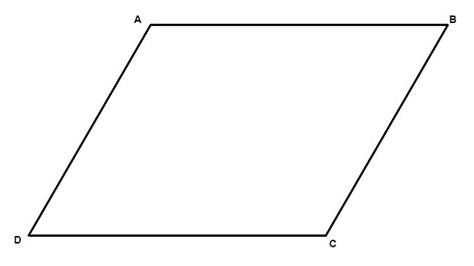There are six important properties of parallelograms to know:
1. Opposite sides are congruent (AB = DC).
2. Opposite angels are congruent (D = B).
3. Consecutive angles are supplementary (A + D = 180°).
4. If one angle is right, then all angles are right.
5. The diagonals of a parallelogram bisect each other.
6. Each diagonal of a parallelogram separates it into two congruent triangles.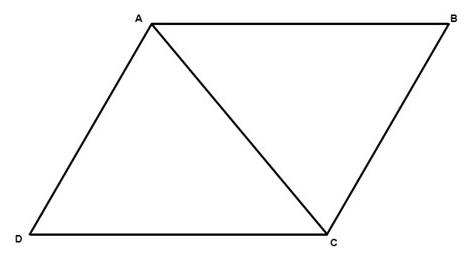△ACD ≅ △ABC
If we have a parallelogram where all sides are congruent then we have what is called a rhombus. The properties of parallelograms can be applied on rhombi.
If we have a quadrilateral where one pair and only one pair of sides are parallel then we have what is called a trapezoid. The parallel sides are called bases while the nonparallel sides are called legs. If the legs are congruent we have what is called an isosceles trapezoid.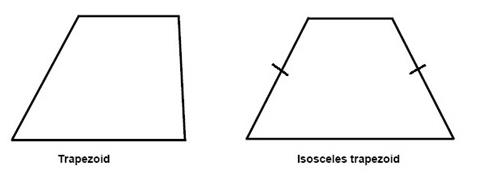In an isosceles trapezoid the diagonals are always congruent. The median of a trapezoid is parallel to the bases and is one-half of the sum of measures of the bases.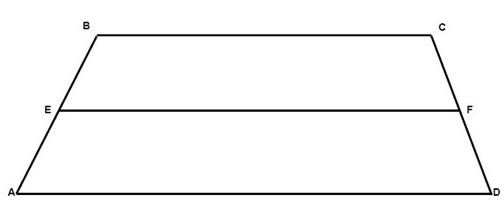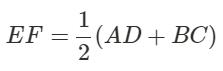Offer running on EduRev: Apply code STAYHOME200 to get INR 200 off on our premium plan EduRev Infinity!

40 docs

,

,

,

,

,

,

,

,

,

,

,

,

,

,

,

,

,

,

,

,

,

;